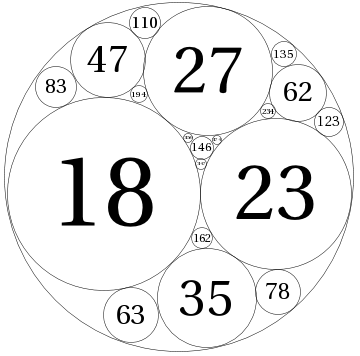arminstraub.com

# Apollonian circle packings in Mathematica

Today, I have been playing a little bit with Apollonian circle packings. Here is the code I wrote in Mathematica to visualize such packings (see below for an example).

```ACPInitialConfiguration[{x1_, x2_, x3_}] :=
Module[{x0, c0, c1, c2, c3},
c1 := 0;
c2 := x2/x1 + 1;
c3 := x3/x1 + (x2 - x1)/(x1 + x2) +
2 I Sqrt[(x1 x2 + x2 x3 + x3 x1)/(x1 + x2)^2];
x0 := x1 + x2 + x3 - 2 Sqrt[x1 x2 + x2 x3 + x3 x1];
c0 := c1 + c2 + c3 - 2 Sqrt[c1 c2 + c2 c3 + c3 c1];
{{x0, c0}, {x1, c1}, {x2, c2}, {x3, c3}}];
ACPNextConfigurations[xc_] :=
Table[Append[Drop[xc, {n}],
2 Total[#] - 3 #[[n]] & /@ Transpose[xc]], {n, 1, 4}];
ACPConfigurations[xc_, n_] :=
NestList[Flatten[ACPNextConfigurations /@ #, 1] &, {xc}, n];
ACPCircle[{x_, c_}, x0_] := Module[{pt = {Re[c/x], Im[c/x]}},
Join[{Circle[pt, 1/x]},
If[x > 0, {Text[Style[x, FontSize -> Scaled[.5 Abs[x0]/x]],
pt]}, {}]]];
ACPCirclePacking[xx_, n_] := Module[{xc, x0},
xc = ACPInitialConfiguration[xx];
x0 = xc[[1, 1]];
Graphics[
Flatten[ACPCircle[#, x0] & /@
Union[Flatten[ACPConfigurations[xc, n], 2]]]]];```
With the above you may visualize a circle packing:
`ACPCirclePacking[{18, 23, 27}, 2]`
The first 3-tuple are the integral curvatures of three kissing circles such that the inscribed fourth circle has integral curvature as well. The last parameter (here 2) specifies the level up to which the circles are included in the visualization.

## Example